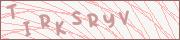# Bez tytułu

z m7, 4 lata temu, napisane w Plain Text, wyświetlone 243 razy.
Udostępnij
Pobierz wklejkę lub Pokaż surowy tekst1. PING 145.239.82.252 (145.239.82.252) 56(84) bytes of data.
2. 64 bytes from 145.239.82.252: icmp_seq=1 ttl=52 time=9.82 ms
3. 64 bytes from 145.239.82.252: icmp_seq=2 ttl=52 time=9.86 ms
4. 64 bytes from 145.239.82.252: icmp_seq=3 ttl=52 time=8.97 ms
5. 64 bytes from 145.239.82.252: icmp_seq=4 ttl=52 time=9.34 ms
6. 64 bytes from 145.239.82.252: icmp_seq=5 ttl=52 time=9.87 ms
7. 64 bytes from 145.239.82.252: icmp_seq=6 ttl=52 time=9.14 ms
8. 64 bytes from 145.239.82.252: icmp_seq=7 ttl=52 time=9.90 ms
9. 64 bytes from 145.239.82.252: icmp_seq=8 ttl=52 time=9.81 ms
10. 64 bytes from 145.239.82.252: icmp_seq=9 ttl=52 time=12.8 ms
11. 64 bytes from 145.239.82.252: icmp_seq=10 ttl=52 time=9.83 ms
12. 64 bytes from 145.239.82.252: icmp_seq=11 ttl=52 time=9.79 ms
13. 64 bytes from 145.239.82.252: icmp_seq=12 ttl=52 time=10.9 ms
14. 64 bytes from 145.239.82.252: icmp_seq=13 ttl=52 time=9.71 ms
15. 64 bytes from 145.239.82.252: icmp_seq=14 ttl=52 time=9.76 ms
16. 64 bytes from 145.239.82.252: icmp_seq=15 ttl=52 time=9.83 ms
17.

# odpowiedź "Bez tytułu"

Tutaj możesz odpowiedzieć na wklejkę z góry